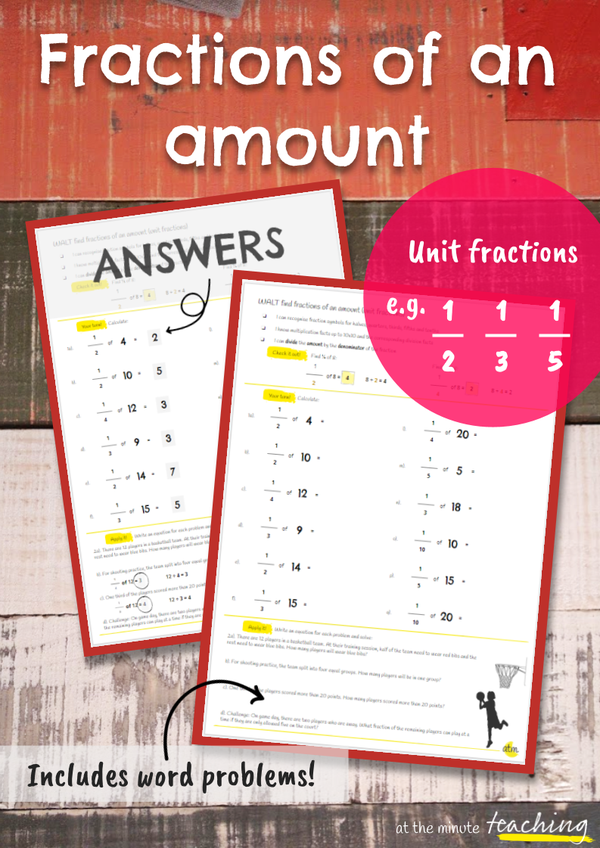#### attheminute teaching

Be purposeful teachers
Who are in control
Feel inspired
And know we've done enough.Kath
Teaching/Maths/Fractions of an amount - Unit fractions worksheet

# Fractions of an amount - Unit fractions worksheet## £0.50GBP

#### Description

In this worksheet, students will calculate fractions of an amount using division. All of the questions are with unit fractions e.g. 1/4 of 12 with denominators of 2, 3, 4, 5, 10 and 12.

• Maths

#### Details

In this worksheet, students will calculate fractions of an amount using division. All of the questions are with unit fractions e.g. 1/4 of 12 with denominators of 2, 3, 4, 5, 10 and 12.

A WALT and success criteria/key mathematical knowledge are included at the top of the worksheet. There are 12 practice questions and 4 word problems which students need to write out a matching equation for before solving.

Answers are included as well as grayscale versions of the question and answer sheets.

### Other resources you might like:

#### attheminute teaching

Be purposeful teachers
Who are in control
Feel inspired
And know we've done enough.Kath